Assess the Modeling Effects of PSS and Governor on Voltage Stability of Power System | OMICS International
Journal of Electrical & Electronic Systems

# Assess the Modeling Effects of PSS and Governor on Voltage Stability of Power System

Amir Sharifian*

Department of Electrical Engineering, Ahrar Institute of Technology and Higher Education, P.O.Box 41931-63584, Rasht, Iran

*Corresponding Author:
Amir Sharifian
Department of Electrical Engineering
Ahrar Institute of Technology and Higher Education
P.O.Box 41931-63584, Rasht, Iran
Tel: +98-13-33424373
Fax: +98-13-33424256
E-mail: [email protected]

Received Date: September 03, 2016; Accepted Date: September 16, 2016; Published Date: September 23, 2016

Citation: Sharifian A (2016) Assess the Modeling Effects of PSS and Governor on Voltage Stability of Power System. J Electr Electron Syst 5: 199. doi: 10.4172/2332-0796.1000199

Copyright: © 2016 Sharifian A. This is an open-access article distributed under the terms of the Creative Commons Attribution License, which permits unrestricted use, distribution, and reproduction in any medium, provided the original author and source are credited.

Visit for more related articles at Journal of Electrical & Electronic Systems

#### Abstract

In this paper, we assess the modeling effects of PSS and governor on voltage stability of power system by applied accurate dynamic model of power system. This model consists of the detailed models for the synchronous machines, automatic voltage regulators (AVRs), Prime mover and speed governor, Power system stabilizer (PSS) is used for voltage stability assessment. Voltage stability assessment is done by calculation of voltage stability margin (VSM). The VSM is an index that describes distance between the current operation state point and the maximum voltage stability limit point. The IEEE 14-bus standard test system is used for simulation. The obtained results from preformed simulation show effects of PSS and governor on voltage stability.

#### Keywords

Voltage stability assessment; Voltage stability margin; Power system modeling

#### Introduction

Due to open market of electricity, economic reasons and environmental constraints the modern power systems are utilized closer to their stability limits and the voltage stability problem has become a major challenge in the utilization of power systems. Hence, the on-line voltage instability assessment has a pivotal contribution in protecting and management of power systems [1,2]. The voltage stability is described capability of a power system to preserve both transferred power and voltage controllable during different contingencies. Voltage instability leads to uncontrollable voltage decrease in a considerable part of the power system. Voltage collapse occurs when the power system is heavily loaded and cannot preserve its generation and transmission scheme.

Various methods have been presented for evaluation the voltage stability problem. They are mainly organized into two discrete categories encompassing static method (such as L-index and modal analysis) and dynamic method (such as time domain simulation) . In the static methods, the steady state power flow model of the power systems is used to evaluate voltage stability. This method is very fast and Practical in online application, but its obtained results is not reliable and accurate as shown in . In the dynamic method [5-7], dynamic model of power system is employed to the voltage stability assessment. Therefore, we need to solve a set of differential and algebraic equations (DAEs) of power system in time domain. Although this approach is very reliable and accurate, it need large calculations time and is not suitable for on-line applications [8-10].

A separate MLP NN has been utilized to monitor online voltage stability for different contingencies . In addition, in order to choose features for training the MLP NN, a regression method has been employed. In order to estimate a long-term voltage stability margin, an artificial neural network (ANN) has been used to the accompaniment of sequential forward selection approach for feature selection . A separate MLP NN is applied to estimate voltage stability margin (VSM) . The load buses voltage magnitudes and active and reactive powers are used the ANN inputs. An artificial feed forward neural network (FFNN) is applied to estimate L-index as an index for voltage stability monitoring in power systems . In this method voltage, real power and reactive power of load and generator buses are utilized to train FFNN. In order to classify the contingencies that result in voltage instability in steady state RBF NN is used . In this method, a correlation coefficient and class reparability index are applied to reduce the number of RBF NN inputs. A single enhanced radial basis function network is utilized to evaluate voltage stability by estimating MW margins for various contingencies . In order to estimate VSM, a RBF NN is employed to the accompaniment of wavelet feature extraction approach . In this method, both Multi-Resolution Wavelet Transform (MRWT) and principle component analysis (PCA) are used to compact the voltage profiles features. Maximum L-index of the load buses is estimated by RBF NN for voltage stability analysis . In this method, pre-contingency state power flows compressed by mutual information method for feature selection are employed as inputs of RBF NN. In ref , in order to assess voltage stability, a MLP NN is utilized to approximate voltage stability margin (VSM). In this method, two procedures inclusive the Gram–Schmidt orthogonalization and ANN-based sensitivity are employed to minimize the number of input variables .

In this paper, we assess the modeling effects of PSS and governor on voltage stability of power system by applied accurate dynamic model of power system. This model consists of the detailed models for the synchronous machines, automatic voltage regulators (AVRs), Prime mover and speed governor, Power system stabilizer (PSS) is used for voltage stability assessment.

Voltage stability assessment is done by calculation of voltage stability margin (VSM). The VSM is an index that describes distance between the current operation state point and the maximum voltage stability limit point. The IEEE 14-bus standard test system is used for simulation. The obtained results from preformed simulation show effects of PSS and governor on voltage stability.

In summary, the innovations of this paper are listed as follows:

• The modeling effects of PSS and governor on voltage stability is evaluated by applied accurate dynamic model of power system consists of the detailed models for the synchronous machines, automatic voltage regulators (AVRs), Prime mover and speed governor, Power system stabilizer (PSS).

• Voltage stability assessment is done by VSM index.

The rest of paper is classified as follows:

Section 4 defines power system model and equations. Section 5 introduces VSM index that is used to assess voltage stability. Section 6 describes the simulation results and discussion and finally, conclusions are outlined in Section 7.

#### Formulation of Multi-Machine Power System with SVC

this section, details modeling of the multi-machine power system with SVC are described. It is assumed that the power system has m busses and n generators. It is mentioned that the assumed power system is simulated by using the software tool PSAT (Power System Analysis Toolbox) . The notations of parameters in power system equations are described in Appendix 1.

Synchronous machine model

In this paper, we apply the two-axis model for description the synchronous machines. The ith synchronous machine fourth-order differential equations are formulated as follows [20-25]: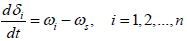(1)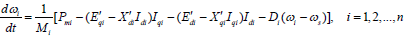(2)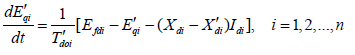(3)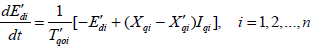(4)

The equation between power network and machine voltages is described as follows: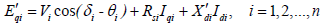(5)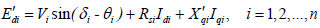(6)

The ith machine currents Idi and Iqi can be obtained as follows: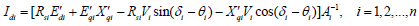(7)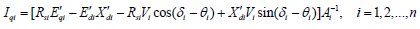(8)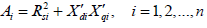(9)

Automatic voltage regulator

The IEEE model 1 is applied for the excitation system (Figure 1). The mathematical formulation of the AVR can be written as follows [20-25]: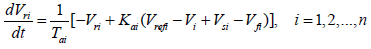(10)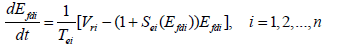(11)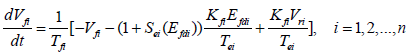(12)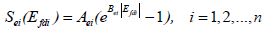(13)

Prime mover and speed governor

The block diagram of a simplified prime mover and speed governor [24,25]. The mathematical formulation can be expressed as follows (Figure 2):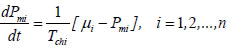(14)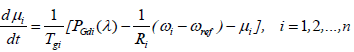(15)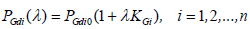(16)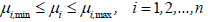(17)

It should be noted that the value of KGi in Eq. (16), can be determined by EDC, AGC or other system operating practice as varies.

Power system stabilizer (PSS)

The PSS is an additional control block that is added to the AVR in order to enhance the system stability [20,21]. A PSS uses stabilizing feedback signals such as shaft speed, terminal frequency and/or power to correct the input signal of the AVR (Figure 3).

SVC

The SVC is applied to maintain or control bus voltage [23,26]. The popular structure of SVC is a parallel combination of thyristor controlled reactor (TCR) with fixed capacitor (Figure 4).

In addition to the main job of the SVC, it is maybe used to damp system oscillations, as denoted by “SVC-sig” (Figure 5).

Network power equations

The power network equations can be described as follows :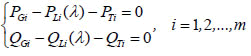(18)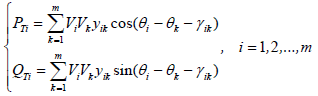(19)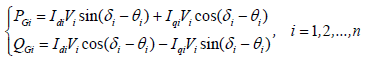(20)

PGi and QGi are primarily obtained by the intrinsic characteristics of the AVR regulations and the speed governor. The values of PLi(λ) and QLi(λ) for different load scenarios can be described as follows: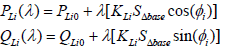(21)

Where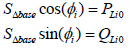(22)

Substituting Eq. (22) in Eq. (21), we have;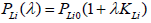(23)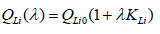(24)

Notice that the values of KLi, KGi, and ?i can be uniquely determined for every bus.

Test system

In this paper an IEEE standard 14-bus test system is applied for voltage stability assessment (Figure 6). The IEEE 14 Bus Test Case represents a portion of the American Electric Power System (in the Midwestern US). This electric network is constituted of 14 buses and 5 generators (at buses No. 1, 2, 3, 6 and 8) injecting their powers for a system nourishing 11 loads through 20 lines of transportation with 11 loads totaling 259 MW and 73.5 MVAR at base case. In addition, this system has 1 static VAR compensator (SVC) that is connected at bus 4.

#### Voltage Stability Assessment

Nowadays, the voltage stability problem is one of the fundamental challenges in design and exploitation of modern power systems. The important factors causing voltage instability are load characteristics, the inability of the power system to generate the demanded reactive power, characteristics of reactive compensation devices and the action of voltage control devices and the generator reactive power limits. Voltage instability leads to uncontrollable voltage decrease in some bus of power system. There are many methods for voltage stability assessment. In general, these methods can be divided into dynamic methods and static methods. In this paper we combine both of dynamic method and static method to voltage stability accurate assessment [27,28].

Voltage stability margin (VSM)

The Voltage stability margin (VSM) is a practical index for voltage stability assessment. The VSM index is defined as the distance from the current operating point to the voltage collapse point according to the system loading parameter λ. It should be noted that in first step, the saddle-node bifurcation (SNB) point or nose point should be located for computing the VSM index. In this paper the continuation methods [24,29,30] is applied to compute the SNB point (Figure 7).

The VSM index is computed using a continuation power flow method. The VSM index is defined as: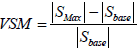(25)

For a power system with m buses, the VSM index can be computed as: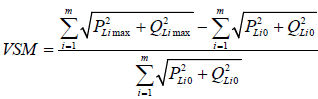(26)

Where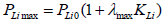(27)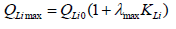(28)

The continuation methods that is applied to compute the VSM index, is precisionist and strong.

#### Results and Discussion

In this section, we evaluate effects of power system modeling on voltage stability margin (VSM). The P-V curve is used for achievement to this aim. It should be noted that, The P-V curve is drawn for PQ buses which load is connected to it.

None of the previous works did not evaluate the effects of PSS and governor modeling on voltage stability margin. In this paper, four different cases of power system modeling are assumed to evaluate effects of power system modeling on voltage stability margin. For 4 assumed cases, the P-V curve is drawn by simulation of power system equations, and then the value of voltage stability margin is computed (Table 1).

Case number Used model for power system modeling
1 Static model for power system
2 Dynamic model of power system includes the 4th order dynamic model for the synchronous machines with automatic voltage regulators (AVRs).
3 Dynamic model of power system includes the 4th order dynamic model for the synchronous machines with automatic voltage regulators (AVRs) and Prime mover and speed governor.
4 Dynamic model of power system includes the 4th order dynamic model for the synchronous machines with automatic voltage regulators (AVRs), Prime mover and speed governor and Power system stabilizer (PSS).

Table 1: Used model for power system modelling.

It should be noted that, in this section, in order to better evaluation of power system modeling effects on voltage stability margin; the connected SVC at bus 4 is disregard.

Case study 1

In this case the static model of power system is used. Therefore, the effects of power system dynamics are ignored.

According to the Figure 8, the computed value of VSM for this case is equal to 2.8286.

Case study 2

In this case the dynamic model of power system includes the 4th order dynamic model for the synchronous machines with automatic voltage regulators (AVRs) is applied.

According to Figure 9, the computed value of VSM for this case is equal to 1.7118. It should be noted that in this case the value of VSM has decreased in compared to case study 1.

Case study 3

In this case the modeling effects of Prime mover and speed governor on voltage stability margin are assessed. For achievement to this purpose, the effects of Prime mover and speed governor are modeled for generators connected at buses 1 and 2. It should be noted that, the generators connected at the buses 3, 6 and 8 are synchronous condensers.

According to Figure 10, the computed value of VSM for this case is equal to 1.7086. It should be noted that in this case the value of VSM has decreased in compared to case study 2.

Case study 4

In this case the modeling effects of Power system stabilizer (PSS) on voltage stability margin are assessed. For achievement to this purpose, the effect of Power system stabilizer (PSS) is modeled for generator connected at buses 1.

According to Figure 11, the computed value of VSM for this case is equal to 1.7119. It should be noted that in this case the value of VSM has increased in compared to case study 3.

In order to better assessment of simulations results, the value of VSM and simulation time associated to four assumed case study are compared together (Table 2).

Case number Value of VSM Simulation time
1 2.8286 2.1494 s
2 1.7118 3.5343 s
3 1.7086 4.0845 s
4 1.7119 4.5202 s

Table 2: The value of VSM and simulation time associated to four assumed case study.

According to Table 2, the obtained value of VSM and simulation time for four assumed case study are different. The simulation time has increased by modeling more details of a power system. On the other hand, modeling more details of power system leads to more accurate calculation of VSM value.

Nowadays, modeling effects of PSS on voltage stability assessment cannot be overlooked because all generators of a practical power system are equipped to PSS. Therefore, the presented model of power system in case study 4 is an operational model for voltage stability assessment. This model is highly accurate and reliable but its simulation is very time-consuming. In this paper proposed model of power system in case study 4 is used to assess voltage stability.

#### Conclusion

In this paper, we assess the modeling effects of PSS and governor on voltage stability of power system by applied accurate dynamic model of power system. This model consists of the detailed models for the synchronous machines, automatic voltage regulators (AVRs), Prime mover and speed governor, Power system stabilizer (PSS) is used for voltage stability assessment.

Voltage stability assessment is done by calculation of voltage stability margin (VSM). The obtained results from preformed simulation show effects of PSS and governor on voltage stability.

#### References

Select your language of interest to view the total content in your interested language

### Article Usage

• Total views: 9821
• [From(publication date):
September-2016 - Jan 22, 2020]
• Breakdown by view type
• HTML page views : 9597Can't read the image? click here to refresh